Weekly and monthly graphs for monitoring crime patterns (SPSS)

I was recently asked for some code to show how I created the charts in my paper, Tables and Graphs for Monitoring Crime Patterns (Pre-print can be seen here).

I cannot share the data used in the paper, but I can replicate them with some other public data. I will use calls for service publicly available from Burlington, VT to illustrate them.

The idea behind these time-series charts are not for forecasting, but to identify anomalous patterns – such as recent spikes in the data. (So they are more in line with the ideas behind control charts.) Often even in big jurisdictions, one prolific offender can cause a spike in crimes over a week or a month. They are also good to check more general trends as well, to see if crimes have had more slight, but longer term trends up or down.

For a preview, we will be making a weekly time series chart: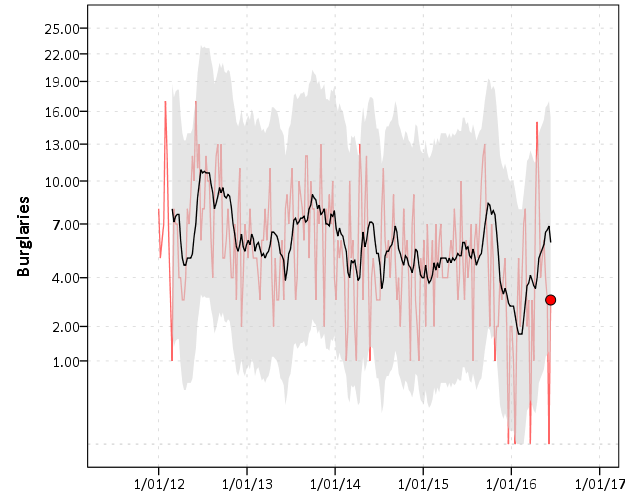In the weekly chart the red line is the actual data, the black line is the average of the prior 8 weeks, and the grey band is a Poisson confidence interval around that prior moving average. The red dot is the most recent week.

And we will also be making a monthly seasonal chart: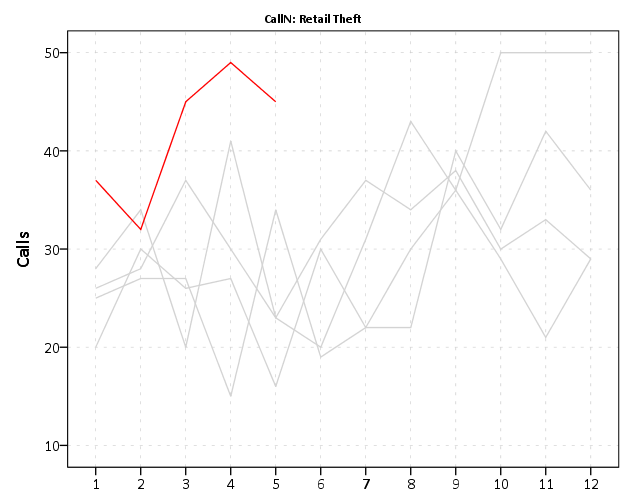The red line is the counts of calls per month in the current year, and the lighter grey lines are prior years (here going back to 2012).

So to start, I saved the 2012 through currently 6/20/2016 calls for service data as a csv file. And here is the code to read in that incident level data.

*Change this to where the csv file is located on your machine.
FILE HANDLE data /NAME = "C:\Users\andrew.wheeler\Dropbox\Documents\BLOG\Tables_Graphs".
GET DATA  /TYPE=TXT
/FILE="data\Calls_for_Service_Dashboard_data.csv"
/ENCODING='UTF8'
/DELCASE=LINE
/DELIMITERS=","
/QUALIFIER='"'
/ARRANGEMENT=DELIMITED
/FIRSTCASE=2
/DATATYPEMIN PERCENTAGE=95.0
/VARIABLES=
AlcoholRelated AUTO
Area AUTO
CallDateTime AUTO
CallType AUTO
Domv AUTO
DayofWeek AUTO
DrugRelated AUTO
EndDateTime AUTO
GeneralTimeofDay AUTO
IncidentNumber AUTO
LocationType AUTO
MentalHealthRelated AUTO
MethodofEntry AUTO
Month AUTO
PointofEntry AUTO
StartDateTime AUTO
Street AUTO
Team AUTO
Year AUTO
/MAP.
CACHE.
EXECUTE.
DATASET NAME CFS.

First I will be making the weekly chart. What I did when I was working as an analyst was make a chart that showed the recent weekly trends and to identify if the prior week was higher than you might expect it to be. The weekly patterns can be quite volatile though, so I smoothed the data based on the average of the prior eight weeks, and calculated a confidence interval around that average count (based on the Poisson distribution).

As a start, we are going to turn our date variable, CallDateTime, into an SPSS date variable (it gets read in as a string, AM/PM in date-times are so annoying!). Then we are going to calculate the number of days since some baseline – here it is 1/1/2012, which is Sunday. Then we are going to calculate the weeks since that Sunday. Lastly we select out the most recent week, as it is not a full week.

*Days since 1/1/2012.
COMPUTE #Sp = CHAR.INDEX(CallDateTime," ").
COMPUTE CallDate = NUMBER(CHAR.SUBSTR(CallDateTime,1,#Sp),ADATE10).
COMPUTE Days = DATEDIFF(CallDate,DATE.MDY(1,1,2012),"DAYS").
COMPUTE Weeks = TRUNC( (Days-1)/7 ).
FREQ Weeks /FORMAT = NOTABLE /STATISTICS = MIN MAX.
SELECT IF Weeks < 233.

Here I do weeks since a particular date, since if you do XDATE.WEEK you can have not full weeks. The magic number 233 can be replaced by sometime like SELECT IF Weeks < (\$TIME - 3*24*60*60). if you know you will be running the syntax on a set date, such as when you do a production job. (Another way is to use AGGREGATE to figure out the latest date in the dataset.)

Next what I do is that when you use AGGREGATE in SPSS, there can be missing weeks with zeroes, which will mess up our charts. There end up being 22 different call-types in the Burlington data, so I make a base dataset (named WeekFull) that has all call types for each week. Then I aggregate the original calls for service dataset to CallType and Week, and then I merge the later into the former. Finally I then recode the missings intos zeroes.

*Make sure I have a full set in the aggregate.
FREQ CallType.
AUTORECODE CallType /INTO CallN.
*22 categories, may want to collapse a few together.
INPUT PROGRAM.
LOOP #Weeks = 0 TO 232.
LOOP #Calls = 1 TO 22.
COMPUTE CallN = #Calls.
COMPUTE Weeks = #Weeks.
END CASE.
END LOOP.
END LOOP.
END FILE.
END INPUT PROGRAM.
DATASET NAME WeekFull.

*Aggregate number of tickets to weeks.
DATASET ACTIVATE CFS.
DATASET DECLARE WeekCalls.
AGGREGATE OUTFILE='WeekCalls'
/BREAK Weeks CallN
/CallType = FIRST(CallType)
/TotCalls = N.

*Merge Into WeekFull.
DATASET ACTIVATE WeekFull.
MATCH FILES FILE = *
/FILE = 'WeekCalls'
/BY Weeks CallN.
DATASET CLOSE WeekCalls.
*Missing are zero cases.
RECODE TotCalls (SYSMIS = 0)(ELSE = COPY).

Now we are ready to calculate our statistics and make our charts. First we create a date variable that represents the beginning of the week (for our charts later on.) Then I use SPLIT FILE and CREATE to calculate the prior moving average only within individiual call types. The last part of the code calculates a confidence interval around prior moving average, and assumes the data is Poisson distributed. (More discussion of this is in my academic paper.)

DATASET ACTIVATE WeekFull.
COMPUTE WeekBeg = DATESUM(DATE.MDY(1,1,2012),(Weeks*7),"DAYS").

*Moving average of prior 8 weeks.
SORT CASES BY CallN Weeks.
SPLIT FILE BY CallN.
CREATE MovAv = PMA(TotCalls,8).
*Calculating the plus minus 3 Poisson intervals.
COMPUTE #In = (-3/2 + SQRT(MovAv)).
DO IF #In >= 0.
COMPUTE LowInt = #In**2.
ELSE.
COMPUTE LowInt = 0.
END IF.
COMPUTE HighInt = (3/2 + SQRT(MovAv))**2.
EXECUTE.

If you rather use the inverse of the Poisson distribution I have notes in the code at the end to do that, but they are pretty similar in my experience. You also might consider (as I mention in the paper), rounding fractional values for the LowInt down to zero as well.

Now we are ready to make our charts. The last data manipulation is to just put a flag in the file for the very last week (which will be marked with a large red circle). I use EXECUTE before the chart just to make sure the variable is available. Finally I keep the SPLIT FILE on, which produces 22 charts, one for each call type.

IF Weeks = 232 FinCount = TotCalls.
EXECUTE.

*Do a quick look over all of them.
GGRAPH
/GRAPHDATASET NAME="graphdataset" VARIABLES=WeekBeg TotCalls MovAv LowInt HighInt FinCount MISSING=VARIABLEWISE
/GRAPHSPEC SOURCE=INLINE.
BEGIN GPL
SOURCE: s=userSource(id("graphdataset"))
DATA: WeekBeg=col(source(s), name("WeekBeg"))
DATA: TotCalls=col(source(s), name("TotCalls"))
DATA: MovAv=col(source(s), name("MovAv"))
DATA: LowInt=col(source(s), name("LowInt"))
DATA: HighInt=col(source(s), name("HighInt"))
DATA: FinCount=col(source(s), name("FinCount"))
SCALE: pow(dim(2), exponent(0.5))
GUIDE: axis(dim(1))
GUIDE: axis(dim(2), label("Crime Count"))
ELEMENT: line(position(WeekBeg*TotCalls), color(color.red), transparency(transparency."0.4"))
transparency.interior(transparency."0.4"), transparency.exterior(transparency."1"))
ELEMENT: line(position(WeekBeg*MovAv))
ELEMENT: point(position(WeekBeg*FinCount), color.interior(color.red), size(size."10"))
END GPL.
SPLIT FILE OFF.

This is good for the analyst, I can monitor many series. Here is an example the procedure produces for mental health calls:The current value is within the confidence band, so it is not alarmingly high. But we can see that they have been trending up over the past few years. Plotting on the square root scale makes the Poisson count data have the same variance, but a nice thing about the SPLIT FILE approach is that SPSS does smart Y axis ranges for each individual call type.

You can update this to make plots for individual crimes, and here I stuff four different crime types into a small multiple plot. I use a TEMPORARY and SELECT IF statement before the GGRAPH code to select out the crime types I am interested in.

FORMATS TotCalls MovAv LowInt HighInt FinCount (F3.0).
TEMPORARY.
SELECT IF ANY(CallN,3,10,13,17).
GGRAPH
/GRAPHDATASET NAME="graphdataset" VARIABLES=WeekBeg TotCalls MovAv LowInt HighInt FinCount CallN MISSING=VARIABLEWISE
/GRAPHSPEC SOURCE=INLINE.
BEGIN GPL
PAGE: begin(scale(900px,600px))
SOURCE: s=userSource(id("graphdataset"))
DATA: WeekBeg=col(source(s), name("WeekBeg"))
DATA: TotCalls=col(source(s), name("TotCalls"))
DATA: MovAv=col(source(s), name("MovAv"))
DATA: LowInt=col(source(s), name("LowInt"))
DATA: HighInt=col(source(s), name("HighInt"))
DATA: FinCount=col(source(s), name("FinCount"))
DATA: CallN=col(source(s), name("CallN"), unit.category())
COORD: rect(dim(1,2), wrap())
SCALE: pow(dim(2), exponent(0.5))
GUIDE: axis(dim(1))
GUIDE: axis(dim(2), start(1), delta(3))
GUIDE: axis(dim(3), opposite())
GUIDE: form.line(position(*,0),color(color.lightgrey),shape(shape.half_dash))
ELEMENT: line(position(WeekBeg*TotCalls*CallN), color(color.red), transparency(transparency."0.4"))
transparency.interior(transparency."0.4"), transparency.exterior(transparency."1"))
ELEMENT: line(position(WeekBeg*MovAv*CallN))
ELEMENT: point(position(WeekBeg*FinCount*CallN), color.interior(color.red), size(size."10"))
PAGE: end()
END GPL.
EXECUTE.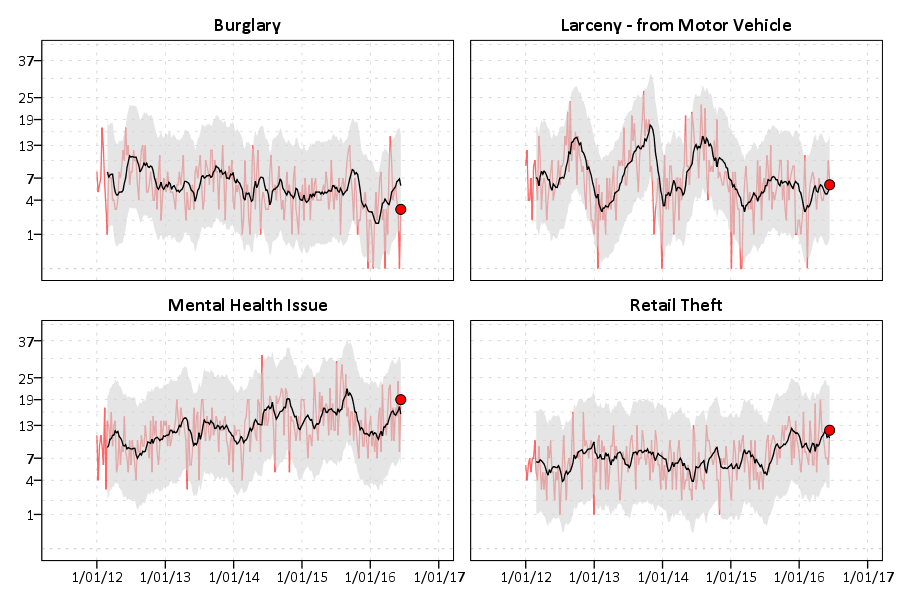You could do more fancy time-series models to create the confidence bands or identify the outliers, (exponential smoothing would be similar to just the prior moving average I show) but this ad-hoc approach worked well in my case. (I wanted to make more fancy models, but I did not let the perfect be the enemy of the good to get at least this done when I was employed as a crime analyst.)

Now we can move onto making our monthly chart. These weekly charts are sometimes hard to visualize with highly seasonal data. So what this chart does is gives each year a new line. Instead of drawing error bars, the past years data show the typical variation. It is then easy to see seasonal ups-and-downs, as well as if the latest month is an outlier.

Getting back to the code — I activate the original calls for service database and then close the Weekly database. Then it is much the same as for weeks, but here I just use calendar months and match to a full expanded set of calls types and months over the period. (I do not care about normalizing months, it is ok that February is only 28 days).

DATASET ACTIVATE CFS.
DATASET CLOSE WeekFull.

COMPUTE Month = XDATE.MONTH(CallDate).
COMPUTE Year = XDATE.YEAR(CallDate).

DATASET DECLARE AggMonth.
AGGREGATE OUTFILE = 'AggMonth'
/BREAK Year Month CallN
/MonthCalls = N.

INPUT PROGRAM.
LOOP #y = 2012 TO 2016.
LOOP #m = 1 TO 12.
LOOP #call = 1 TO 22.
COMPUTE CallN = #call.
COMPUTE Year = #y.
COMPUTE Month = #m.
END CASE.
END LOOP.
END LOOP.
END LOOP.
END FILE.
END INPUT PROGRAM.
DATASET NAME MonthAll.

MATCH FILES FILE = *
/FILE = 'AggMonth'
/BY Year Month CallN.
DATASET CLOSE AggMonth.

Next I select out the most recent month of the date (June 2016) since it is not a full month. (When I originally made these charts I would normalize to days and extrapolate out for my monthly meeting. These forecasts were terrible though, even only extrapolating two weeks, so I stopped doing them.) Then I calculate a variable called Current – this will flag the most recent year to be red in the chart.

COMPUTE MoYr = DATE.MDY(Month,1,Year).
FORMATS MoYr (MOYR6) Year (F4.0) Month (F2.0).
SELECT IF MoYr < DATE.MDY(6,1,2016).
RECODE MonthCalls (SYSMIS = 0)(ELSE = COPY).

*Making current year red.
COMPUTE Current = (Year = 2016).
FORMATS Current (F1.0).

SORT CASES BY CallN MoYr.
SPLIT FILE BY CallN.

*Same thing with the split file.
GGRAPH
/GRAPHDATASET NAME="graphdataset" VARIABLES=Month MonthCalls Current Year
/GRAPHSPEC SOURCE=INLINE.
BEGIN GPL
SOURCE: s=userSource(id("graphdataset"))
DATA: Month=col(source(s), name("Month"), unit.category())
DATA: MonthCalls=col(source(s), name("MonthCalls"))
DATA: Current=col(source(s), name("Current"), unit.category())
DATA: Year=col(source(s), name("Year"), unit.category())
GUIDE: axis(dim(1))
GUIDE: axis(dim(2), label("Calls"), start(0))
GUIDE: legend(aesthetic(aesthetic.color.interior), null())
SCALE: cat(aesthetic(aesthetic.color.interior), map(("0",color.lightgrey),("1",color.red)))
ELEMENT: line(position(Month*MonthCalls), color.interior(Current), split(Year))
END GPL.

You can again customize this to be individual charts for particular crimes or small multiples. You can see in the example at the beginning of the post Retail thefts are high for March, April and May. I was interested to examine overdoses, as the northeast (and many parts of the US) are having a problem with heroin at the moment. In the weekly charts they are so low of counts it is hard to see any trends though.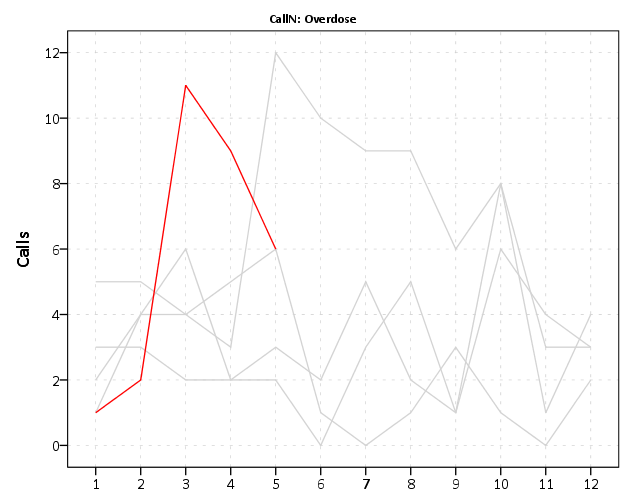We can see that overdoses were high in March. The other highest line are months in 2015, so it looks like a problem here in Burlington, but it started around a year ago.

For low counts of crime (say under 20 per month) seasonality tends to be hard to spot. For crimes more frequent though you can often see pits and peaks in summer and winter. It is not universal that crimes increase in the summer though. For ordinance violations (and ditto for Noise complaints) we can see a pretty clear peak in September. (I don’t know why that is, there is likely some logical explanation for it though.)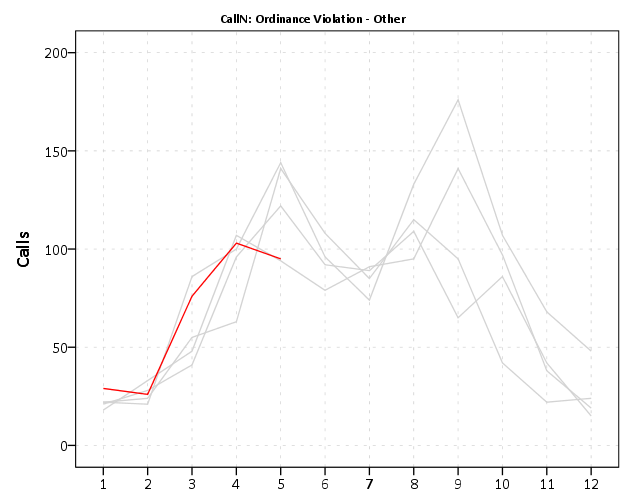My main motivation to promote these is to replace terrible CompStat tables of year-over-year percent changes. All of these patterns I’ve shown are near impossible to tell from tables of counts per month.

Finally if you want to export your images to place into another report, you can use:

OUTPUT EXPORT /PNG IMAGEROOT = "data\TimeGraphs.png".

PNG please – simple vector graphics like these should definately not be exported as jpegs.

Here is a link to the full set of syntax and the csv data to follow along. I submitted to doing an hour long training session at the upcoming IACA conference on this, so hopefully that gets funded and I can go into this some more.

Shape, color, and pattern constants in SPSS charts

I have a version of the SPSS (Statistics) Version 24 GPL reference guide bookmarked here. The reference guide is great to skim through and see what is possible in SPSS charts – especially the set of examples on pages 329 to 411.

On page 413 they also give a set of constant colors, shapes, and texture patterns you can use in charts. Colors you can also specify in RGB scale, but it is often convenient to just say color.red or color.pink etc. Shapes and patterns for practical purposes you have to choose among the constants. (Technically in the chart template you can edit the cycle styles, and change a circle to an ellipse for example, or change the points for a dash pattern, but this would be painful for anything besides a few constants.)

Here is a handy reference guide to actually visualize those constants. Many you can guess what they look like, but the colors are more subtle. Who knew there were differences between tomato, salmon, and pink! (The tomato is more like tomato soup color.)

Here are the color constants (you can open the chart in a new tab to see a larger image):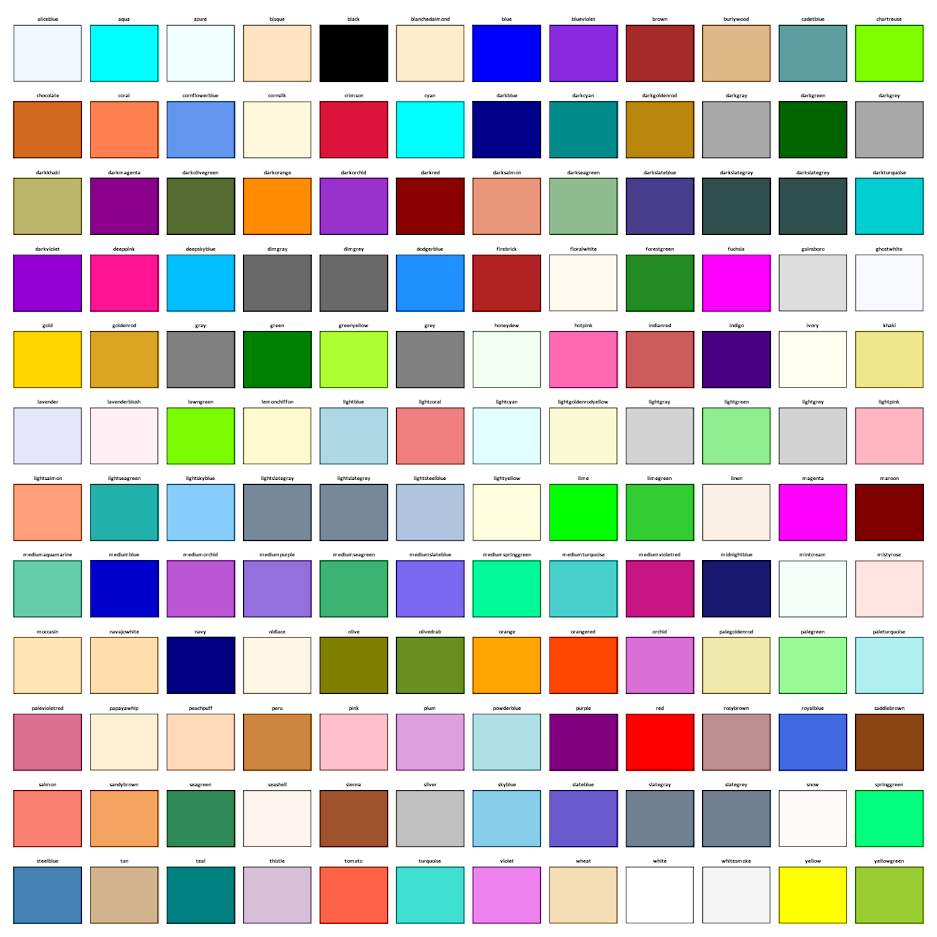The shape constants: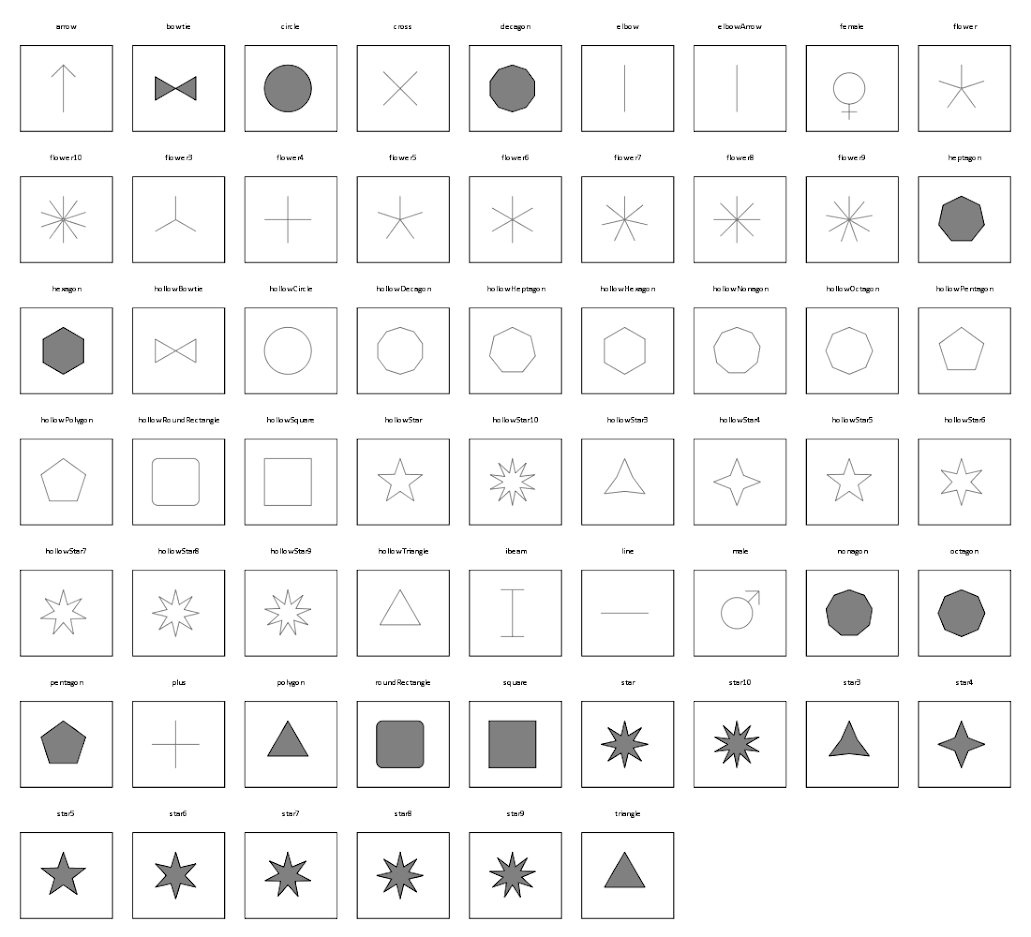The elbow and the elbowArrow do not look correct – but will take some more time to investigate. The others look ok to me though. (The number of sides and star points appear to me to be something you can also manipulate in the chart template cycles, if for some reason you want a hendecagon).

And here are the pattern constants. I plot them with a grey filled interior – you can see some specifically only have an outline and always have a transparent fill: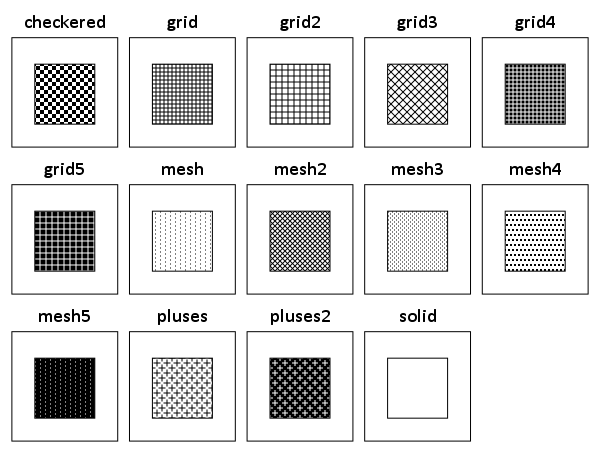Here is code to replicate the charts, and here is a PDF to download with the constants. The colors and shapes are hard to read because they are squeezed in so small, but you can zoom into the PDF and read the categories. I haven’t used dashed lines ever, so I omit those constants here. (Patterns I use pretty rarely, but I have used them if there are only two categories.)

A useful change for the colors would be sorting in a logical order. They are just currently in alphabetical. I am too lazy though to convert the colors to a colorspace and sort them though. (Maybe converting the PDF to SVG would do the trick easy enough though.)

On overlapping error bars in charts

Andrew Gelman posted an example graph the other day in a blog post which showed trends over time in measures of smiling in high school yearbook photos.Surprisingly, Andrew did not make a comment on the error bars in the graph. Error bars with cross hairs are often distracting in the plot. In the example graph it is quite bad, in that they perfectly overlap, so the ends are very difficult to disentangle. Here I will suggest some alternatives.

I simulated data that approximately captures the same overall trends, and replicated the initial chart in SPSS.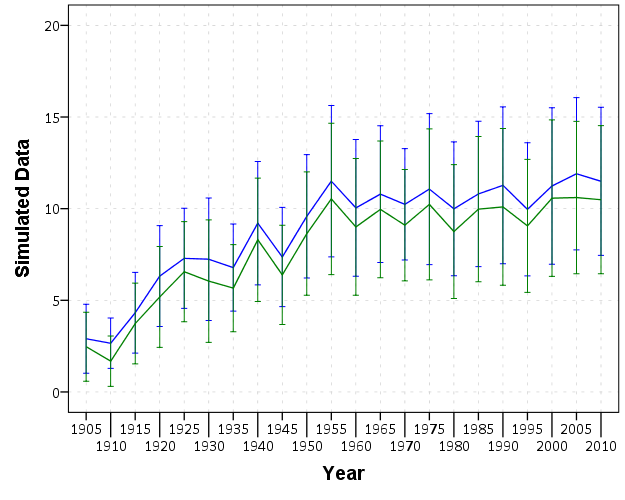First, a simple solution with only two groups is to use semi-transparent areas instead of the error bars.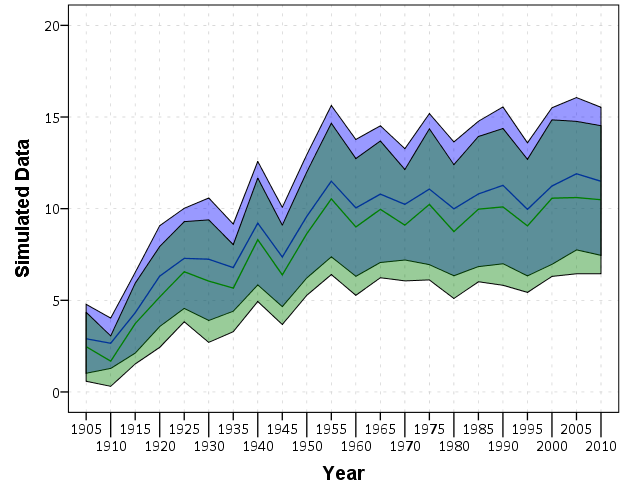This makes it quite easy to see the overlap and non-overlap of the two groups. This will even print out nice in black-white. In the end, this chart is over-complicated by separating out genders. Since each follow the same trend, with females just having a constant level shift over the entire study period, there is not much point in showing each in a graph. A simpler solution would just pool them together (presumably the error bars would be smaller by pooling as well). The advice here still applies though, and the areas are easier to viz. than the discontinuous error bars.

For more complicated plots with more groups, I would suggest doing small multiples.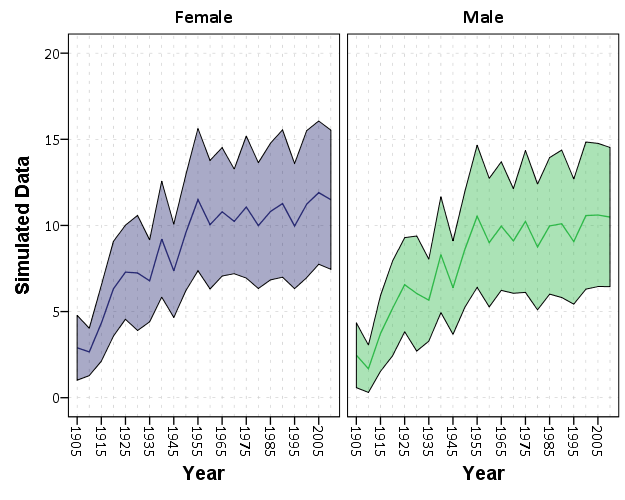While it is harder now to see the exact overlap between groups, we can at least visually assess the trends within each group quite well. In the original it is quite a bit of work to figure out the differences between groups and keep the within group comparisons straight. Since the trends are so simple it is not impossible, but with more variable charts it would be quite a bit of work.

For instances in which a trend line is not appropriate, you can dodge the individual error bars on the x-axis so that they do not perfectly overlap. This is the same principle as in clustered bar charts, just with points and error bars instead of bars.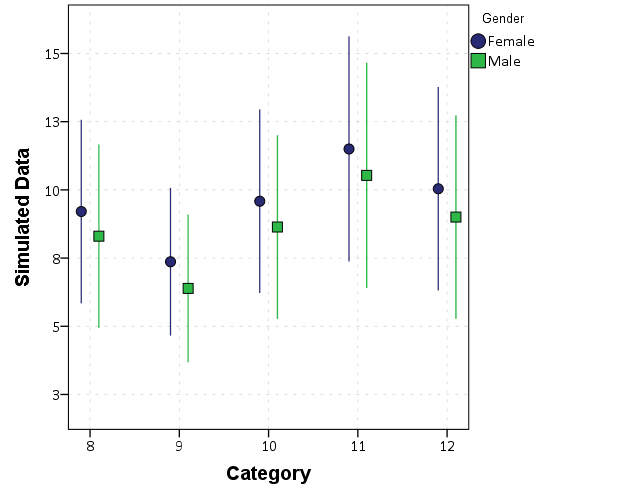Here I like using just the straight lines (a tip taken from Andrew Gelman). The serif part of the I beam like error bars I find distracting, and make it necessary to separate the lines further. Using just the lines you can pack many more into a small space, like caterpillar plots of many random effects.

Here is a copy of the SPSS syntax used to generate these graphs.

Maps in inline GPL statements (SPSS)

Here I will go through an example of using inline GPL statements to import map backgrounds in SPSS charts. Here you can download the data and code to follow along with this post. This is different than using maps via VIZTEMPLATE, as I will show.

Note you can also use the graphboard template chooser to make some default maps, but I’ve never really learned how to make them on my own. For example, say I want to map that sets both the color and the transparency of areas based on different attributes. This is not possible with the current selection of map templates that comes with SPSS (V22).

But I figured out some undocumented ways to import maps into inline GPL code, and you can get pretty far with just the possibilities available within the grammar of graphics.

The data I will be using is a regular grid of values across DC. What I calculated was the hour of the day with the most Robberies over along time period (2011 through 2015 data) using a weighted average approach synonymous with geographically weighted regression. Don’t take this too seriously though, as there appears to be some errors in the time fields for the historical DC crime data.

So below I first define a handle to where my data is stored, recode the hour field into a smaller set of bins, and then make a scatterplot.

FILE HANDLE data /NAME = "C:\Users\andrew.wheeler\Dropbox\Documents\BLOG\Inline_Maps_GGRAPH".

GET FILE = "data\MaxRobHour.sav".
DATASET NAME MaxRob.

*Basic Scatterplot.
FREQ HourEv.
RECODE HourEv (0 THRU 3 = 1)(11 THRU 19 = 2)(ELSE = COPY) INTO HourBin.
VALUE LABELS HourBin
1 '0 to 3'
2 '11 to 19'.

DATASET ACTIVATE MaxRob.
* Chart Builder.
GGRAPH
/GRAPHDATASET NAME="graphdataset" VARIABLES=XMetFish[LEVEL=SCALE] YMetFish[LEVEL=SCALE] HourBin
MISSING=LISTWISE REPORTMISSING=NO
/GRAPHSPEC SOURCE=INLINE.
BEGIN GPL
SOURCE: s=userSource(id("graphdataset"))
DATA: XMetFish=col(source(s), name("XMetFish"))
DATA: YMetFish=col(source(s), name("YMetFish"))
DATA: HourBin=col(source(s), name("HourBin"), unit.category())
GUIDE: axis(dim(1), label("XMetFish"))
GUIDE: axis(dim(2), label("YMetFish"))
GUIDE: legend(aesthetic(aesthetic.color.exterior), label("HourBin"))
ELEMENT: point(position(XMetFish*YMetFish), color.exterior(HourBin))
END GPL.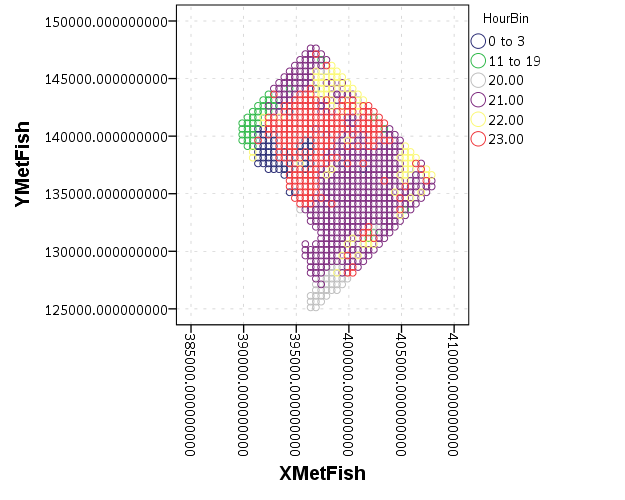We can do quite a bit to make this map look nicer. Here I change:

• make the aspect ratio 1 to 1, and set the map limits
• get rid of the X and Y axis (the particular projected coordinates make no difference)
• make a nice set of colors based on a ColorBrewer palatte and map the color to the interior of the point

And below that is the map it produces.

*Making chart nice, same aspect ratio, colors, drop x & y.
FORMATS HourBin (F2.0).
GGRAPH
/GRAPHDATASET NAME="graphdataset" VARIABLES=XMetFish[LEVEL=SCALE] YMetFish[LEVEL=SCALE] HourBin
MISSING=LISTWISE REPORTMISSING=NO
/GRAPHSPEC SOURCE=INLINE.
BEGIN GPL
SOURCE: s=userSource(id("graphdataset"))
DATA: XMetFish=col(source(s), name("XMetFish"))
DATA: YMetFish=col(source(s), name("YMetFish"))
DATA: HourBin=col(source(s), name("HourBin"), unit.category())
COORD: rect(dim(1,2), sameRatio())
GUIDE: axis(dim(1), null())
GUIDE: axis(dim(2), null())
GUIDE: legend(aesthetic(aesthetic.color.exterior), label("HourBin"))
SCALE: linear(dim(1), min(389800), max(408000))
SCALE: linear(dim(2), min(125000), max(147800))
SCALE: cat(aesthetic(aesthetic.color.interior), map(("1",color."810f7c"),("2",color."edf8fb"),("20",color."bfd3e6"),("21",color."9ebcda"),
("22",color."8c96c6"),("23",color."8856a7")))
ELEMENT: point(position(XMetFish*YMetFish), color.interior(HourBin))
END GPL.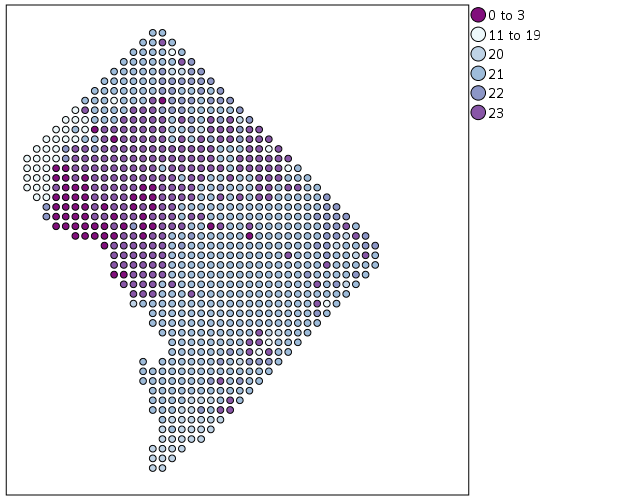So that is not too shabby a map for just plain SPSS. Now it is a bit hard to vizualize the patterns though, because the surface has needless discontinuities because of the circles. We can use squares as the shape and just do some experimentation to figure out the size needed to fill up each grid cell. Also pro-tip when making choropleth maps, with many areas often light outlines look nicer than black ones.

*Alittle nicer, squares, no outline.
GGRAPH
/GRAPHDATASET NAME="graphdataset" VARIABLES=XMetFish[LEVEL=SCALE] YMetFish[LEVEL=SCALE] HourBin
MISSING=LISTWISE REPORTMISSING=NO
/GRAPHSPEC SOURCE=INLINE.
BEGIN GPL
SOURCE: s=userSource(id("graphdataset"))
DATA: XMetFish=col(source(s), name("XMetFish"))
DATA: YMetFish=col(source(s), name("YMetFish"))
DATA: HourBin=col(source(s), name("HourBin"), unit.category())
COORD: rect(dim(1,2), sameRatio())
GUIDE: axis(dim(1), null())
GUIDE: axis(dim(2), null())
GUIDE: legend(aesthetic(aesthetic.color.exterior), label("HourBin"))
SCALE: linear(dim(1), min(389800), max(408000))
SCALE: linear(dim(2), min(125000), max(147800))
SCALE: cat(aesthetic(aesthetic.color.interior), map(("1",color."810f7c"),("2",color."edf8fb"),("20",color."bfd3e6"),("21",color."9ebcda"),
("22",color."8c96c6"),("23",color."8856a7")))
ELEMENT: point(position(XMetFish*YMetFish), color.interior(HourBin), shape(shape.square), size(size."9.5"),
transparency.exterior(transparency."1"))
END GPL.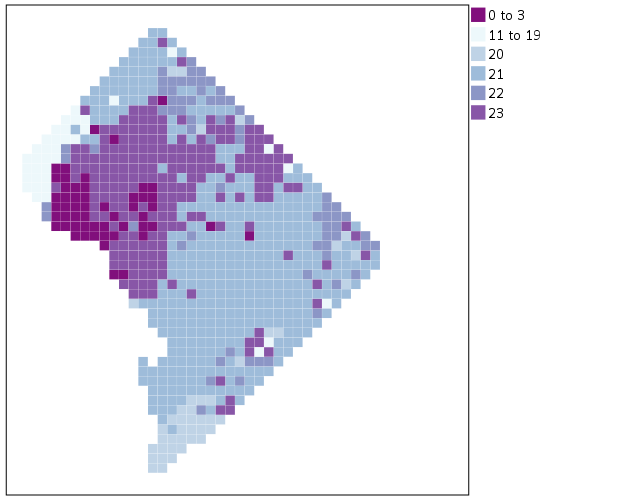Again looking pretty good for just a map in plain SPSS. With the larger squares it is easier to clump together areas with similar patterns for the peak robbery time. The city never sleeps in Georgetown it appears. A few of the polygons though are very hard to see on the edge of DC though, so we will add in the outline. See the SOURCE: mapsrc, DATA: lon*lat, and the ELEMENT: polygon lines for how this is done. The “DCOutline.smz” is the map template file created by SPSS.

*Now include the outline.
GGRAPH
/GRAPHDATASET NAME="graphdataset" VARIABLES=XMetFish[LEVEL=SCALE] YMetFish[LEVEL=SCALE] HourBin
MISSING=LISTWISE REPORTMISSING=NO
/GRAPHSPEC SOURCE=INLINE.
BEGIN GPL
SOURCE: s=userSource(id("graphdataset"))
DATA: XMetFish=col(source(s), name("XMetFish"))
DATA: YMetFish=col(source(s), name("YMetFish"))
DATA: HourBin=col(source(s), name("HourBin"), unit.category())
SOURCE: mapsrc = mapSource(file("C:\\Users\\andrew.wheeler\\Dropbox\\Documents\\BLOG\\Inline_Maps_GGRAPH\\DCOutline.smz"))
DATA: lon*lat = mapVariables(source(mapsrc))
COORD: rect(dim(1,2), sameRatio())
GUIDE: axis(dim(1), null())
GUIDE: axis(dim(2), null())
GUIDE: legend(aesthetic(aesthetic.color.exterior), label("HourBin"))
SCALE: linear(dim(1), min(389800), max(408000))
SCALE: linear(dim(2), min(125000), max(147800))
SCALE: cat(aesthetic(aesthetic.color.interior), map(("1",color."810f7c"),("2",color."edf8fb"),("20",color."bfd3e6"),("21",color."9ebcda"),
("22",color."8c96c6"),("23",color."8856a7")))
ELEMENT: point(position(XMetFish*YMetFish), color.interior(HourBin), shape(shape.square), size(size."9.5"),
transparency.exterior(transparency."1"))
ELEMENT: polygon(position(lon*lat))
END GPL.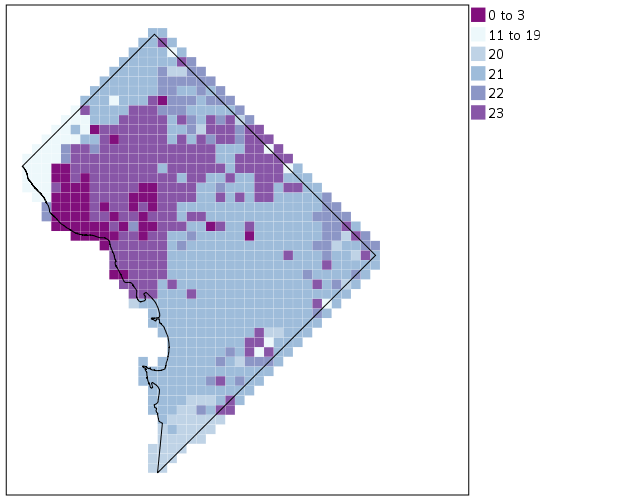Now we have a bit more of a reference. The really late at night area appears to be north of Georgetown. The reason I figured this was even possible is that although mapSource is not documented in the GPL reference guide, there is an example using it with the project function (see page 194).

Now, if I were only making one map this isn’t really much of a help – I would just export the data values, make it in ArcGIS and be done with it. But, one of the things hard to do in GIS is make small multiple maps. That is something we can do fairly easily in stat. software though. For an example, here I make a random map to compare with the observed patterns. The grammar automatically recognizes lon*lat*Type and replicates the background outline across each panel. Also I change the size of the overall plot using PAGE statements. I just typically experiment until it looks nice.

*Can use the outline to do small multiples.
COMPUTE HourRand = TRUNC(RV.UNIFORM(0,24)).
RECODE HourRand (0 THRU 3 = 1)(4 THRU 19 = 2)(ELSE = COPY).
VARSTOCASES
/MAKE Hour FROM HourBin HourRand
/INDEX Type.
VALUE LABELS Type 1 'Observed' 2 'Random'.

*Small multiple.
GGRAPH
/GRAPHDATASET NAME="graphdataset" VARIABLES=XMetFish YMetFish Hour Type
MISSING=LISTWISE REPORTMISSING=NO
/GRAPHSPEC SOURCE=INLINE.
BEGIN GPL
PAGE: begin(scale(1000px,500px))
SOURCE: s=userSource(id("graphdataset"))
DATA: XMetFish=col(source(s), name("XMetFish"))
DATA: YMetFish=col(source(s), name("YMetFish"))
DATA: Hour=col(source(s), name("Hour"), unit.category())
DATA: Type=col(source(s), name("Type"), unit.category())
SOURCE: mapsrc = mapSource(file("C:\\Users\\andrew.wheeler\\Dropbox\\Documents\\BLOG\\Inline_Maps_GGRAPH\\DCOutline.smz"))
DATA: lon*lat = mapVariables(source(mapsrc))
COORD: rect(dim(1,2), sameRatio(), wrap())
GUIDE: axis(dim(1), null())
GUIDE: axis(dim(2), null())
GUIDE: axis(dim(3), opposite())
GUIDE: legend(aesthetic(aesthetic.color.exterior), label("HourBin"))
SCALE: linear(dim(1), min(389800), max(408000))
SCALE: linear(dim(2), min(125000), max(147800))
SCALE: cat(aesthetic(aesthetic.color.interior), map(("1",color."810f7c"),("2",color."edf8fb"),("20",color."bfd3e6"),("21",color."9ebcda"),
("22",color."8c96c6"),("23",color."8856a7")))
ELEMENT: point(position(XMetFish*YMetFish*Type), color.interior(Hour), shape(shape.square), size(size."8"),
transparency.exterior(transparency."1"))
ELEMENT: polygon(position(lon*lat*Type))
PAGE: end()
END GPL.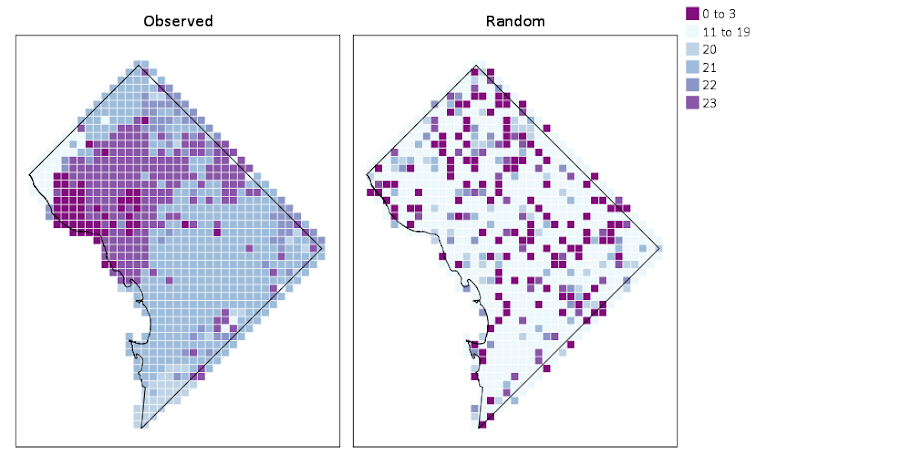We can see that this extreme amount of clustering is clearly not random.

This example works out quite nice because the micro level areas are a regular grid, so I can simulate a choropleth map look by just using square point markers. Unfortunately, I was not able to figure out how to map areas to merge a map file and an id like you can in VIZTEMPLATE. You can see some of my attempts in the attached code. You can however have multiple mapSource statements, so you could import say a street network, rivers and parks and map a nice background map right in SPSS. Hopefully IBM updates the documentation so I can figure out how to make a choropleth map in inline GPL statements.

Turning SPSS data into Python data

Previously I blogged about how to take Python data and turn it back into SPSS data. Here we are going to do the opposite — turn SPSS data into Python objects. First to start out we will make a simple dataset of three variables.

DATA LIST Free /X Y (2F1.0) Z (A1).
BEGIN DATA
1 2 A
4 5 B
7 8 C
END DATA.
DATASET NAME Test.
EXECUTE.

To import this data into Python, we need to import the spss class of functions, which then you can read cases from the active dataset using the Cursor attribute. Here is an example of grabbing all of the cases.

*Importing all of the data.
BEGIN PROGRAM Python.
import spss
dataCursor = spss.Cursor()
AllData = dataCursor.fetchall()
dataCursor.close()
print AllData
END PROGRAM.

What this then prints out is ((1.0, 2.0, 'A'), (4.0, 5.0, 'B'), (7.0, 8.0, 'C')), a set of nested tuples. You can also just grab one case by replacing dataCursor.fetchall() with dataCursor.fetchone(), in which case it will just return one tuple.

To only grab particular variables from the list, you can pass a set of indices in the spss.Cursor object. Remember, Python indices start at zero, so if you want the first and second variables in the dataset, you need to grab the 0 and 1 indices.

*Only grabbing certain variables.
BEGIN PROGRAM Python.
dataNum = spss.Cursor([0,1])
spNumbers = dataNum.fetchall()
dataNum.close()
print spNumbers
END PROGRAM.

This subsequently prints out ((1.0, 2.0), (4.0, 5.0), (7.0, 8.0)). When grabbing one variable, you may want just a list of the objects instead of the nested tuples. Here I use list comprehension to turn the resulting tuples for the Z variable into a nice list.

*Converting to a nice list.
BEGIN PROGRAM Python.
dataAlp = spss.Cursor()
spAlp = dataAlp.fetchall()
dataAlp.close()
spAlp_list = [i for i in spAlp] #convert to nice list
print spAlp
print spAlp_list
END PROGRAM.

The first print object is (('A',), ('B',), ('C',)), but the second is ['A', 'B', 'C'].

The above code works fine if you know the position of the variable in the file, but if the position can change this won’t work. Here is a one liner to get the variable names of the active dataset and plop them in a list.

*Way to get SPSS variable names.
BEGIN PROGRAM Python.
varList = [spss.GetVariableName(i) for i in range(spss.GetVariableCount())]
print varList
END PROGRAM.

Now if you have your list of variable names you want, you can figure out the index value. There are two ways to do it, iterate over the list of variable names in the dataset, or iterate over the list of your specified variables. I do the latter here (note this will result in an error if you supply a variable name not in the dataset).

*Find the indices of specific variables.
BEGIN PROGRAM Python.
LookVars = ["X","Z"]
VarInd = [varList.index(i) for i in LookVars]
print VarInd
END PROGRAM.

Now you can just supply VarInd above to the argument for spss.Cursor to grab those variables. Here I wrapped it all up in a function.

*Easy function to use.
BEGIN PROGRAM Python.
import spss
def AllSPSSdat(vars):
if vars == None:
varNums = range(spss.GetVariableCount())
else:
allvars = [spss.GetVariableName(i) for i in range(spss.GetVariableCount())]
varNums = [allvars.index(i) for i in vars]
data = spss.Cursor(varNums)
pydata = data.fetchall()
data.close()
return pydata
END PROGRAM.

You can either supply a list of variables or None, in the latter case all of the variables are returned.

BEGIN PROGRAM Python.
MyDat = AllSPSSdat(vars=["Y","Z"])
print MyDat
END PROGRAM.

This set of nested tuples is then pretty easy to convert to other Python objects. Panda’s dataframes, Numpy arrays, and NetworkX objects are all one liners. Here is turning the entire dataset into a panda’s data frame.

*Turn into pandas data frame.
BEGIN PROGRAM Python.
import pandas as pd
MyDat = AllSPSSdat(vars=None)
allvars = [spss.GetVariableName(i) for i in range(spss.GetVariableCount())]
PanDat = pd.DataFrame(list(MyDat),columns=allvars)
print PanDat
END PROGRAM.

Which prints out.

X  Y  Z
0  1  2  A
1  4  5  B
2  7  8  C

Using local Python objects in SPSSINC TRANS – examples with network statistics

When using SPSSINC TRANS, you have a wider array of functions to compute on cases in SPSS. Within the local session, you can create your own python functions within a BEGIN PROGRAM and END PROGRAM block. In SPSSINC TRANS you pass in the values in the current dataset, but you can also create functions that use data in the local python environment as well. An example use case follows in which you create a network in the local python environment using SPSS data, and then calculate several network statistics on the nodes. Here is a simple hierarchical network dataset that signifies managers and subordinates in an agency.

*Edge list.
DATA LIST FREE / Man Sub (2F1.0).
BEGIN DATA
1 2
2 3
2 4
3 5
3 6
4 7
4 8
END DATA.
DATASET NAME Boss.

We can subsequently turn this into a NetworkX graph with the code below. Some of my prior SPSS examples using NetworkX had a bit more complicated code using loops and turning the SPSS dataset into the network object. But actually the way SPSS dumps the data in python (as a tuples nested within a list) is how the add_edges_from function expects it in NetworkX, so no looping required (and it automatically creates the nodes list from the edge data).

BEGIN PROGRAM Python.
import networkx as nx
import spss, spssdata

alldata = spssdata.Spssdata().fetchall()  #get SPSS data
G = nx.DiGraph()                          #create empty graph
print G.nodes()
END PROGRAM.

Note now that we have the graph object G in the local python environment for this particular SPSS session. We can then make our own functions that references G, but takes other inputs. Here I have examples for the geodesic distance between two nodes, closeness and degree centrality, and the average degree of the neighbors.

BEGIN PROGRAM Python.
#path distance
def geo_dist(source,target):
return nx.shortest_path_length(G,source,target)
#closeness centrality
def close_cent(v):
return nx.closeness_centrality(G,v)
#degree
def deg(v):
return G.degree(v)
#average degree of neighbors
def avg_neideg(v):
return nx.average_neighbor_degree(G,nodes=[v])[v]
END PROGRAM.

Here is the node list in a second SPSS dataset that we will calculate the mentioned statistics on. For large graphs, this is nice because you can select out a smaller subset of nodes and only worry about the calculations on that subset. For a crime analysis example, I may be monitoring a particular set of chronic offenders, and I want to calculate how close every arrested person within the month is to the set of chronic offenders.

DATA LIST FREE / Employ (F1.0).
BEGIN DATA
1
2
3
4
5
6
7
8
END DATA.
DATASET NAME Emp.
DATASET ACTIVATE Emp.

Now we have all the necessary ingredients to calculate our network statistics on these nodes. Here are examples of using SPSSINC TRANS to calculate the network statistics in the local SPSS dataset.

*Geodesic distance from 1.
SPSSINC TRANS RESULT=Dist TYPE=0
/FORMULA "geo_dist(source=1.0,target=Employ)".

*closeness centrality.
SPSSINC TRANS RESULT=Cent TYPE=0
/FORMULA "close_cent(v=Employ)".

*degree.
SPSSINC TRANS RESULT=Deg TYPE=0
/FORMULA "deg(v=Employ)".

*Average neighbor degree.
SPSSINC TRANS RESULT=NeighDeg TYPE=0
/FORMULA "avg_neideg(v=Employ)".

Fuzzy matching in SPSS using a custom python function

The other day I needed to conduct propensity score matching, but I was working with geographic data and wanted to restrict the matches to within a certain geographic distance. To do this I used the FUZZY extension command, which allows you to input a custom function. To illustrate I will be using some example data from my dissertation, and the code and data can be downloaded here.

So first lets grab the data and reduce it down a bit to only the variables we will be using. This dataset are street segments and intersections in DC, and the variables are crime, halfway houses, sidewalk cafes, and bars. Note to follow along you need to update the file handle to your machine.

FILE HANDLE save /NAME = "!!!Your Handle Here!!!".
GET FILE = "save\BaseData.sav".
DATASET NAME DC_Data.
SORT CASES BY MarID.

*Reduce the variable list down a bit.
MATCH FILES FILE = * /KEEP  MarID XMeters YMeters OffN1 OffN2 OffN3 OffN4 OffN5 OffN6 OffN7 OffN8 OffN9
TotalCrime HalfwayHouse SidewalkCafe TypeC_D.

Now as a quick illustration, I am going to show a propensity score analysis predicting the location of halfway houses in DC – and see if street units with a halfway house are associated with more violence. Do not take this as a serious analysis, just as an illustration of the workflow. The frequency shows there are only 9 halfway houses in the city, and the compute statements collapse crimes into violent and non-violent. Then I use PLUM to fit the logistic model predicting the probability of treatment. I use non-violent crimes, sidewalk cafes, and bars as predictors.

FREQ HalfwayHouse.
COMPUTE Viol = OffN1 + OffN4 + OffN5 + OffN6.
COMPUTE NonViol = OffN2 + OffN3 + OffN7 + OffN8 + OffN9.

*Fitting logit model via PLUM.
PLUM HalfwayHouse WITH NonViol SidewalkCafe TypeC_D
/CRITERIA=CIN(95) DELTA(0) LCONVERGE(0) MXITER(100) MXSTEP(5) PCONVERGE(1.0E-6) SINGULAR(1.0E-8)
/PRINT=FIT PARAMETER SUMMARY
/SAVE=ESTPROB.

The model is very bad, but we can see that sidewalk cafes are never associated with a halfway house! (Again this is just an illustration – don’t take this as a serious analysis of the effects of halfway houses on crime.) Now we need to make a custom function with which to restrict matches not only based on the probability of treatment, but also based on the geographic location. Here I made a file named DistFun.py, and placed in it the following functions:

#These functions are for SPSS's fuzzy case control matching
import math
#distance under 500, and caliper within 0.02
def DistFun(d,s):
dx = math.pow(d - s,2)
dy = math.pow(d - s,2)
dis = math.sqrt(dx + dy)
p = abs(d - s)
if dis < 500 and p < 0.02:
t = 1
else:
t = 0
return t
#distance over 500, but under 1500
def DistBuf(d,s):
dx = math.pow(d - s,2)
dy = math.pow(d - s,2)
dis = math.sqrt(dx + dy)
p = abs(d - s)
if dis  500 and p < 0.02:
t = 1
else:
t = 0
return t

The FUZZY command expects a function to return either a 1 for a match and 0 otherwise, and the function just takes a fixed set of vectors. The first function DistFun, takes a list where the first two elements are the coordinates, and the last element is the probability of treatment. It then calculates the euclidean distance, and returns a 1 if the distance is under 500 and the absolute distance in propensity scores is under 0.02. The second function is another example if you want matches not too close but not too far away, at a distance of between 500 and 1500. (In this dataset my coordinates are projected in meters.)

Now to make the research reproducible, what I do is save this python file, DistFun.py, in the same folder as the analysis. To make this an importable function in SPSS for FUZZY you need to do two things. 1) Also have the file __init__.py in the same folder (Jon Peck made the comment this is not necessary), and 2) add this folder to the system path. So back in SPSS we can add the folder to sys.path and check that our function is importable. (Note that this is not permanent change to the PATH system variable in windows, and is only active in the same SPSS session.)

*Testing out my custom function.
BEGIN PROGRAM Python.
import sys
sys.path.append("!!!Your\\Path\\Here!!!\\")

import DistFun

#test case
x = [0,0,0.02]
y = [0,499,0.02]
z = [0,500,0.02]
print DistFun.DistFun(x,y)
print DistFun.DistFun(x,z)
END PROGRAM.

Now we can use the FUZZY command and supply our custom function. Without the custom function you could specify the distance in any one dimension on the FUZZ command (e.g. here something like FUZZ = 0.02 500 500), but this produces a box, not a circle. Also with the custom function you can do more complicated things, like my second buffer function. The function takes the probability of treatment along with the two spatial coordinates of the street unit.

*This uses a custom function I made to restrict matches to within 500 meters.
FUZZY BY=EST2_1 XMeters YMeters SUPPLIERID=MarID NEWDEMANDERIDVARS=Match1 Match2 Match3 GROUP=HalfwayHouse CUSTOMFUZZ = "DistFun.DistFun"
EXACTPRIORITY=FALSE
MATCHGROUPVAR=MGroup
/OPTIONS SAMPLEWITHREPLACEMENT=FALSE MINIMIZEMEMORY=TRUE SHUFFLE=TRUE SEED=10.

This takes less than a minute, and in this example provides a full set of matches for all 9 cases (not surprising, since the logistic regression equation predicting halfway house locations is awful). Now to conduct the propensity score analysis just takes alittle more data munging. Here I make a second data of just the matched locations, and then reshape the cases and controls so they are in long format. Then I merge the original data back in.

*Reshape, merge back in, and then conduct outcome analysis.
DATASET COPY PropMatch.
DATASET ACTIVATE PropMatch.
SELECT IF HalfwayHouse = 1.
VARSTOCASES /MAKE MarID FROM MarID Match1 Match2 Match3
/INDEX Type
/KEEP MGroup.

*Now remerge original data back in.
SORT CASES BY MarID.
MATCH FILES FILE = *
/TABLE = 'DC_Data'
/BY MarID.

Now you can conduct the analysis. For example most people use t-tests both for the outcome and to assess balance on the pre-treatment variables.

*Now can do your tests.
T-TEST GROUPS=HalfwayHouse(0 1)
/MISSING=ANALYSIS
/VARIABLES=Viol
/CRITERIA=CI(.95).

One of my next projects will be to use this workflow to conduct fuzzy name matching within and between police databases using custom string distance functions.

Passing arguments to SPSSINC TRANS (2)

Jon Peck made some great comments on my prior post on passing arguments to the SPSSINC TRANS function. Besides advice on that I should be quoting the argument on the FORMULA statement, he gave examples of how you can use the "TO" argument in both passing variables lists within the python formula and assigning variables to the results. Here is a brief example of their use.

First I will be working with a tiny, toy dataset:

DATA LIST FREE / X1 TO X4.
BEGIN DATA
1 2 3 4
5 6 7 8
9 8 7 6
5 4 3 2
END DATA.
DATASET NAME Test.

Now here is a command that returns the second lowest value in a list. (While there are plenty of things you can do in base code, this python code is very simple compared to what you would have to do in vanilla SPSS to figure this out.) In a nutshell you can specify the variable list on the /VARIABLE subcommand (and mix in TO to specify adjacent variables as in most SPSS commands). And then insert these into the python formula by specifying <>.

SPSSINC TRANS RESULT = Second
/VARIABLES X1 TO X4
/FORMULA "sorted([<>])".

In my prior post, I showed how you could do this for the original variables, which would look like /FORMULA "sorted([X1,X2,X3,X4])". Here you can see I’ve specified a set of variables on the VARIABLES subcommand, and inserted them into a list using [<>]. Enclosing <> in brackets produces a list in python. I then sort the list and grab the second element (located at 1, since python uses 0 based indices). You can also mix variables in the dataset and the <> listed on the variables subcommand. See here for an example.

You can also use the TO modifier in making a new set of variables. Here I return the sorted variables X1 TO X4 as a new set of variables S1 TO S4.

SPSSINC TRANS RESULT = S1 TO S4
/VARIABLES X1 TO X4
/FORMULA "sorted([<>])".

In both the prior examples I omitted the TYPE argument, as it defaults to 0 (i.e. a numeric variable returned as a float). But when specifying variable lists of the same type for multiple variables you can simply specify the type one time and the rest of the results are intelligently returned as the same. Here is the same sorted example, except that I return the results each as a string of length 1 as opposed to a numeric value.

SPSSINC TRANS RESULT = A1 TO A4 TYPE = 1
/VARIABLES X1 TO X4
/FORMULA "map(str, sorted([<>]))".

SPSS Predictive Analytics Blog

SPSS had a blog on the old developerworks site, but they’ve given it a bit of a reboot recently. I’ve volunteered to have my old SPSS posts uploaded to the site, and this is what I said I wanted back in 2012; a blogging community related to SPSS. So when blogging about SPSS related topics I will be cross-posting the posts both here and predictive analytics blog. Hopefully the folks at IBM can get more individuals to participate in writing posts.

Passing arguments to SPSSINC TRANS

So I actually bothered to read the help the other day for SPSSINC TRANS, which being generic allows you to use Python functions similar to how COMPUTE statements work, just a bit more general. Two examples of passing arguments I did not know you could do were 1) pass a list as an argument, and 2) pass constants that aren’t SPSS variables to functions. To follow are a few brief examples.

The first is passing a list to a function, and here is a simple example using the Python function sorted().

DATA LIST FREE / X1 X2 X3.
BEGIN DATA
3 2 1
1 0 3
1 1 2
2 2 1
3 0 3
END DATA.
DATASET NAME Test.

SPSSINC TRANS RESULT=S1 S2 S3 TYPE=0
/FORMULA sorted([X1,X2,X3]).

This takes the variables X1 to X3, sorts them, and returns them in a new set of variables S1 to S3. We can also do reverse sorting by passing a constant value of 1 to the reverse function, which acts synonymously with reverse=True.

SPSSINC TRANS RESULT=RS1 RS2 RS3 TYPE=0
/FORMULA sorted([X1,X2,X3],reverse=1).

This is a rather simplistic example, but the action is much simpler in Python than whatever equivalent SPSS code you can come up with. When using the SPSSINC TRANS extension it expects the returned function to simply be a flat list. For this sorting situation though it might be convenient to return the order in which the original value was stored. Here I make a function that returns the indice of the original list, and then flattens the two into sequential order, per this SO answer.

BEGIN PROGRAM Python.
import itertools

def SortList(L,reverse=0):
I = range(1,len(L)+1)
x = sorted(zip(L,I),reverse=reverse)
r = list(itertools.chain.from_iterable(x))
return r

#example use
print SortList(L=[2,1,3])
print SortList(L=[2,1,3],reverse=1)
END PROGRAM.

MATCH FILES FILE = * /DROP S1 TO RS3.

SPSSINC TRANS RESULT= S1 T1 S2 T2 S3 T3 TYPE=0
/FORMULA SortList([X1,X2,X3],reverse=1).

When passing a string constant to a function in SPSSINC TRANS you need to triple quote the string. This makes some of my prior examples of using the Google maps related API’s much simpler. Instead of making variables to pass to the function, you can just triple quote the constants. Also when using the maps API I often have an argument for the API key, but you will get results even without a key (I presume Google just checks the IP address an limits you after so many requests). So for many of my functions you can not worry about making an API key and just pass an empty string. Here is an example from my prior Google distance API post using string constants and no API key.

BEGIN PROGRAM Python.
import urllib, json

#This parses the returned json to pull out the distance in meters and
#duration in seconds, [None,None] is returned is status is not OK
def ExtJsonDist(place):
if place['rows']['elements']['status'] == 'OK':
meters = place['rows']['elements']['distance']['value']
seconds = place['rows']['elements']['duration']['value']
else:
meters,seconds = None,None
return [meters,seconds]

#Takes a set of lon-lat coordinates for origin and destination,
#plus your API key and returns the json from the distance API
def GoogDist(OriginX,OriginY,DestinationX,DestinationY,key):
'?origins=%s,%s'
'&destinations=%s,%s'
'&key=%s') % (OriginY,OriginX,DestinationY,DestinationX,key)
response = urllib.urlopen(MyUrl)
data = ExtJsonDist(jsonData)
return data
END PROGRAM.

*Grab the online data.
DATASET CLOSE ALL.
SPSSINC GETURI DATA
URI="https://dl.dropboxusercontent.com/u/3385251/NewYork_ZipCentroids.sav"
FILETYPE=SAV DATASET=NY_Zips.

*Selecting out only a few.
SELECT IF \$casenum <= 5.
EXECUTE.

SPSSINC TRANS RESULT=Meters Seconds TYPE=0 0
/FORMULA GoogDist(OriginX=LongCent,OriginY=LatCent,DestinationX='''-78.276205''',DestinationY='''42.850721''',key=''' ''').